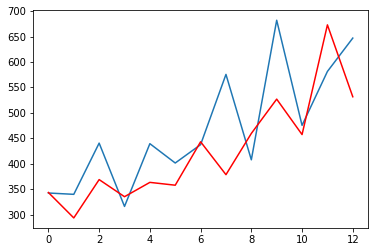Course Content

# Time Series Analysis

1. Time Series: Let's Start

2. Time Series Processing

3. Time Series Visualization

Time Series Analysis

##Autoregressive-Integrated-Moving-Average Model

The last model we will look at is the autoregressive integrated moving average. This model combines autoregression, moving average and differencing technique(this is not the model we discussed above). It is presented as follows:It may look quite complicated, but in reality, look, `AR` - is an autoregressive model with which you are already familiar, `MA` - is a moving average, which implies a linear combination of errors from the past forecast.

ARIMA uses 3 parameters that we must choose ourselves `(p, d, q)`:

`P` - is called the order of autoregression. It is the number of immediately preceding values in the series that are used to predict the value at the present time;

`D` - order of differencing;

`Q` - the order of the moving average. Allows you to set the model error as a linear combination of previously observed error values.

Note

We will consider differencing techniques in the next chapters in more detail

What is the advantage of this model? It can forecast simple non-stationary processes ( more precisely, processes in which mean and covariance changes over time) and is more efficient when working with short-term predictions.

Let's create an ARIMA model using `statsmodels`, for this, and we use the class `ARIMA()`:

The results:Basically, you will use 2 functions: `.forecast()` and `.predict()` The first function is for predictions outside of the dataset (which is why we used a loop in the example above), while the `.predict()` function is used for predictions inside the dataset.

Further, you can experiment with the `p`, `q`, and `d` parameters to get the best results. But even with these parameters, you can see that the model tracks the main trends well.

The code may process for up to 1-minute

Create an ARIMA model and train it on the `pr_air_quality.csv` dataset.
1. Within the `for` loop, create an ARIMA model using the `history` data and assign it to the `model` variable. Then, fit `model` to the data and save it as `model_fit`. Then, make forecasts using fitted `model_fit`.
2. Calculate RMSE: take the square root (`sqrt`) of the mean squared error (calculated using the `test` and `predictions`).
3. Visualize the results: display the `test` values within the first call of the `.plot()` function and `predictions` values within the second call.